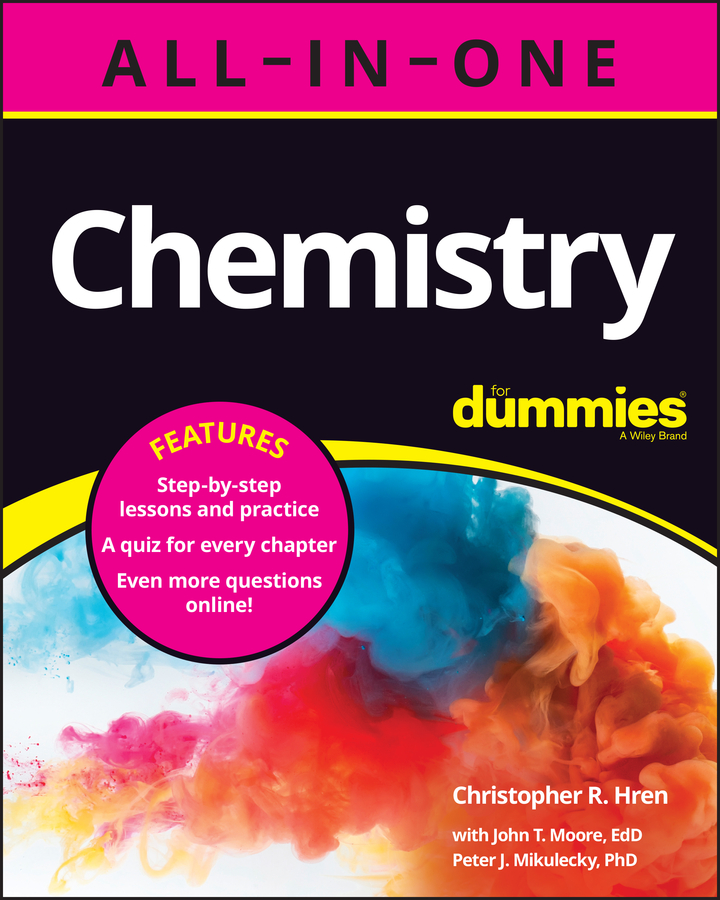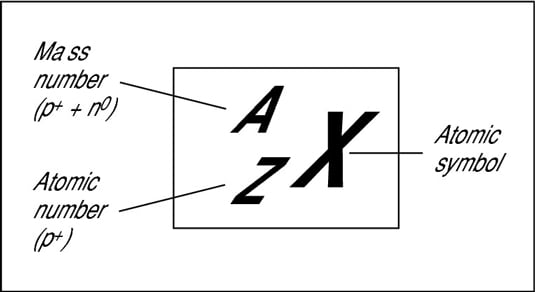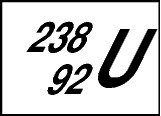##### Chemistry All-in-One For Dummies (+ Chapter Quizzes Online)The nucleus, that dense central core of the atom, contains both protons and neutrons. Electrons are outside the nucleus in energy levels. Protons have a positive charge, neutrons have no charge, and electrons have a negative charge.

A neutral atom contains equal numbers of protons and electrons. But the number of neutrons within an atom of a particular element can vary. Atoms of the same element that have differing numbers of neutrons are called isotopes.

## How chemists describe specific isotopes

The following diagram shows the symbolization chemists use to represent a specific isotope of an element. In this diagram:
• X represents the chemical symbol of the element found on the periodic table.

• Z represents the atomic number.

• A represents the mass number (also called the atomic weight).## A quick example

Suppose you want to represent uranium. You can refer to a periodic table or a list of elements, and find that the symbol for uranium is U, its atomic number is 92, and its mass number is 238.

So, you can represent uranium as shown here:You know that uranium has an atomic number of 92 (number of protons) and mass number of 238 (protons plus neutrons). So if you want to know the number of neutrons in uranium, all you have to do is subtract the atomic number (92 protons) from the mass number (238 protons plus neutrons). The resulting number shows that uranium has 146 neutrons.

But how many electrons does uranium have? Because the atom is neutral (it has no electrical charge), there must be equal numbers of positive and negative charges inside it, or equal numbers of protons and electrons. So there are 92 electrons in each uranium atom.

The nucleus is very, very small and very, very dense when compared to the rest of the atom. Not only is the nucleus very small, but it also contains most of the mass of the atom. In fact, for all practical purposes, the mass of the atom is the sum of the masses of the protons and neutrons.

## Crowded nucleus, nuclear glue

The protons of an atom are all crammed together inside the nucleus. Each proton carries a positive charge, and like charges repel each other. However, forces in the nucleus counteract this repulsion and hold the nucleus together. (Physicists call these forces nuclear glue. But sometimes this “glue” isn’t strong enough, and the nucleus does break apart. This process is called radioactivity.)